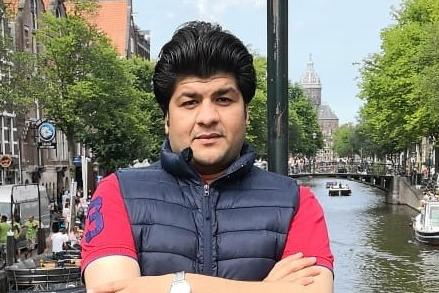Post Date
Jun 3 2023

## Numerical Simulations and Error Analysis of Fractional Non-Newtonian Flows

Year
2022
Supervisor:
Dr. Amer Rasheed
Students:
Mumtaz Khan
MS/PhD
PhD
Reference / Filters
Mathematics

Abstract:

The field of fractional calculus has been exceptionally developing since the inception of the idea in 1695 by Leibniz and L’Hôpital. The mathematical research groups extended their research interests to the fractional derivatives as they can candidly be presumed to generalize the integer-order derivatives. Moreover, very few research studies present logical inferences from the real-world physical phenomena studied through fractional derivatives. The field of fractional calculus has also captured the interest of many researchers from the field of Physics, acoustics, bio engineering, seismology, medical physics, imaging, and stochastics, etc. due to the fact that it can be used to model the non-locality, memory, spatial heterogeneity, and anomalous diffusion inherited in a number of real-world problems. The investigation of non-Newtonian fluid is a significant illustration of fractional calculus when the transport in a viscoelastic non-Newtonian fluid converts into “anisotropic” due to the distribution of fluid density and heterogeneity internal temperature while an external force created by shear flow is applied. Since the non-Newtonian fluids contain intricate multi-term time-fractional dynamical systems having diffusion and time-fractional diffusion terms in addition to a non-linear reaction term, so the conventional research techniques of universal applications and mathematical characterization utilized to investigate the Newtonian fluids are no longer valid. The aforesaid multifarious systems are modeled with the aid of fractional calculus by means of intercalating among the integer orders of differential equations in order to comprehend the non-local association in time and space using a fractional operator. The available techniques in literature cannot determine the exact solutions of coupled viscoelastic non-linear differential equations, and due attention is also not extended to the numerical solutions of atypical non-linear fractional flows. Therefore, in the recent past, several researchers have performed focused studies to discover novel and up-to-date theoretical and numerical techniques to determine solutions of fractional hydro-dynamical systems. Various concerns regarding viscoelastic simulations have been addressed by researchers with the passage of time. These issues may form a basis for restructuring the model in order to develop an efficient numerical scheme. In our thesis, we have taken into consideration only the viscoelastic fluids with different flow formations. Primarily, a structural description of the problem is offered, followed by fundamental laws essential for modeling the presented problem. Further, we have developed an up-to-date model for viscoelastic fluids where various physical effects like a magnetic field, variable thermal heat flux, joule heating phenomenon, etc., are applied over different geometrical surfaces keeping in view their industrial utility. In order to solve the aforesaid fractional viscoelastic fluid model, we have considered computational approaches of Finite difference approximations along with the \$L_1\$ algorithm to discretize the fractional time derivative and Finite Element discretization for spatial variables to determine the solution of fractional viscoelastic models. The benefits of using this model are manifold and will result in an efficient and precise solution with reference to different rheological models and kinds of nonlinearities that occur in the Non-Newtonian systems. We have formulated a code for our proposed numerical scheme and carried out numerical convergence and error analysis intending to establish the validity of the code. To conclude, we are confident enough that this thesis will be a valuable contribution to the studies of computational and fractional calculus over a viscoelastic fluid in addition to the beneficial count in industrial and engineering applications.

Publications:

1.       Mumtaz Khan, Amer Rasheed, Scott-Blair model with unequal diffusivities of chemical species through a Forchheimer medium, Journal of Molecular Liquids, Vol. 341(3),pp. 117351 2021.

2.       Mumtaz Khan, Amer Rasheed, The Space-Time Coupled Fractional Cattaneo-Friedrich Maxwell Model with Caputo Derivatives, International Journal of Applied and Computational Mathematics, Vol. 7(3), 2021.

3.       Mumtaz Khan, Amer Rasheed, Taimoor Salahuddin, Shafqat Ali, Chemically reactive flow of hyperbolic tangent fluid flow having thermal radiation and double stratification embedded in porous medium, Ain Shams Engineering Journal, 12(3) 3209-3216, 2021.

4.       Mumtaz Khan, Amer Rasheed, Exothermic impact on Eyring-Powell nanofluid flow through a heated surface embedded in porous medium with thermal radiation: A numerical study, Physica Scripta, Vol. 96, pp. 035213, 2021.

5.       Mumtaz Khan, Amer Rasheed, Slip velocity and temperature jump effects on molybdenum disulfide MoS2 and silicon oxide SiO2 hybrid nano-fuid near irregular 3D surface, Alexandria Engineering Journal, Vol. 60(1), pp. 1689 - 1701, 2020.